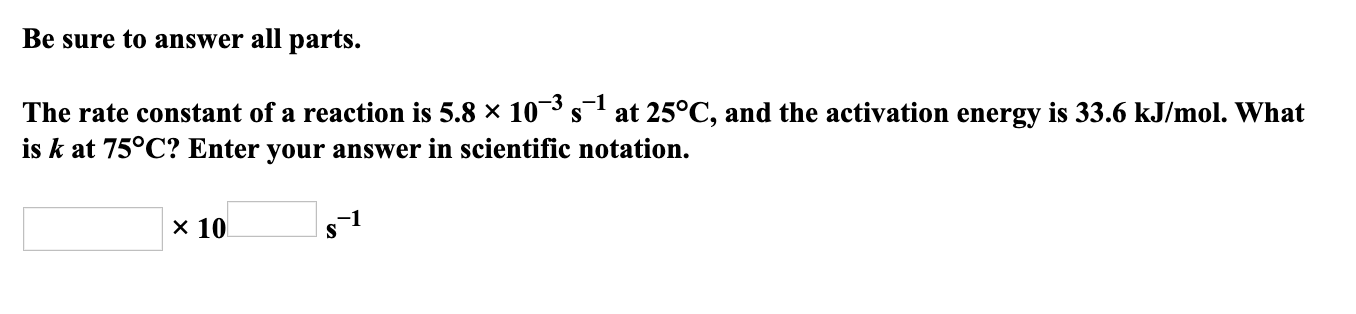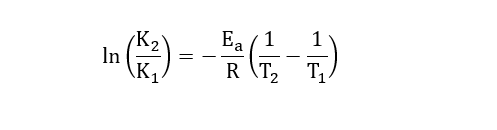# Be sure to answer all parts.The rate constant of a reaction is 5.8 × 10¯³ s at 25°C, and the activation energy is 33.6 kJ/mol. Whatis k at 75°C? Enter your answer in scientific notation.x 10s1

Question
26 views

The rate constant of a reaction is 5.8*10-3s-1 at 25 degrees celcius and the activation energy is 33.6 kJ/mole. What is k at 75 degrees celcius? Enter your answer in scientific notation.help_outlineImage TranscriptioncloseBe sure to answer all parts. The rate constant of a reaction is 5.8 × 10¯³ s at 25°C, and the activation energy is 33.6 kJ/mol. What is k at 75°C? Enter your answer in scientific notation. x 10 s1 fullscreen
check_circle

Step 1

Given:

Temperature T1 = 25 oC = 298 K.

Temperature T2 = 75 oC = 348 K.

Activation energy (Ea) = 33.6 KJ/mol.

Rate Constant at T1 (K1) = 5.8×10-3 s-1.

Rate Constant at T2 (K2) = ?

Step 2

Arrhenius equation is given by,...

### Want to see the full answer?

See Solution

#### Want to see this answer and more?

Solutions are written by subject experts who are available 24/7. Questions are typically answered within 1 hour.*

See Solution
*Response times may vary by subject and question.
Tagged in

### Chemistry